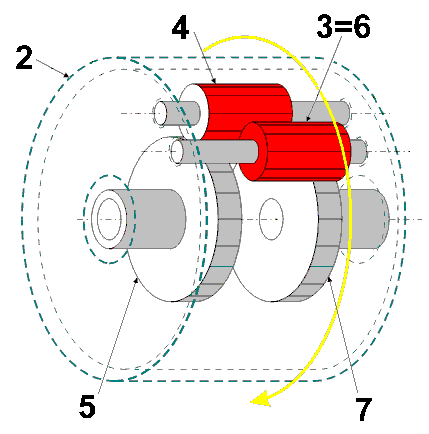# Understanding Gears

To get a hold on the various designs presented in our collection isn't as hard as it may seem on first sight. The most complex feature employed is the differential - at the end of this page you will have mastered it!

## Spur Wheels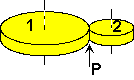Let's start with a simple pair of spur wheels, numbered 1 and 2 in the sketch nearby. They mesh in a single point, called "point of contact" (POC) and marked P in the sketch. We take it for granted that the meshing is perfect, i.e. no teeth are missing or skipped. So, when wheel 1 is turned, a number of teeth passes the POC, which stays in place as long as the shafts bearing the gears stay put. Every tooth passing POC forces one tooth of wheel 2 pass POC as well, which in turn makes wheel 2 turn as well - but in the opposite direction! Pressing this simple fact into an algebraic form, we get
 (1) n1 * rot1 = n2 * rot2 * (-1)   or   n1 * rot1 + n2 * rot2 = 0
where ni is the total number of teeth of wheel i, roti denotes the angle - e.g. measured in turns - wheel i turns and the negative sign is used to describe the direction - positive being CCW usually.
For the first exercise we had held the shafts fixed. Now mentally fix wheel 1 to the table and turn wheel 2 by moving its shaft on a circular path round wheel 1. Does formula (1) still hold true? This depends on the "point of view" (POV): If you define rot as rotation around the shaft measured against a fixed line somewhere on the table, you are stuck as rot1 will be zero for this case. But if you tie a string round both shafts this string will always cross the POC as well. And if you define rot for each gear as angle relative to this string all is fine. Remember, depending on POV the wheel moves (first exercise) or the string moves! So, as a rule:
 (2) Formula (1) is valid only if rot is measured relative to the POC.
As last step imagine that wheel 1 is not fixed to the table but is slowly turning CCW. At the same time wheel 2 is orbiting wheel 1 with the shaft moving CW on a circle as in the previous exercise. What will happen: Strictly adhering to rule (2), the turning of wheel 1 does not have any effect on rot1 nor rot2. Nevertheless it is obviously possible to change the rotational speed of wheel 2 relative to its shaft by changing the turning rate of wheel 1. This "superposition" effect shall be formulated as rule:
 (3) rot = wrot + drot
The "wheel rotation" wrot is measured with both shafts (and by this the POC) staying on a fixed position on the table, while the "displacement rotation" drot is observed when the other (driving) wheel is held completely stationary (i.e. does not turn) relative to the table.

Make sure the three rules given so far are understood. If insecure grab some gear wheels and play with them! Or try your skills with example 1 further down.

## Differential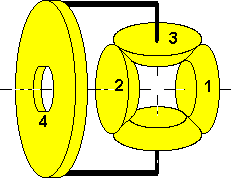Now you are ready to leave the plane and move on to 3D constructions. Look at our generalized differential in the picture nearby. It consists of four shafts: Wheels 1 and 2 have their own shafts brought out to the right and left hand sides. Wheel 3 is wedged in between, meshing with both of them at the same time. Wheel 3's shaft is kinked 90 deg to the left and fixed to wheel 4, which in turn has its own shaft (not drawn). The trick is that if wheel 4 turns, it takes the shaft of wheel 3 with it and vice versa.
With this configuration we have three basic modes of operation:
• Reversal: Block wheel 4. If you turn wheel 1, wheel 3 turns on its (now fixed) shaft and drives wheel 2 in the opposite direction.
• Reduction: Block wheel 2. If you turn wheel 1, wheel 3 is turned but has to roll on the blocked wheel 2 as well. Wheel 3's only possibility is to take its shaft along, by this turning wheel 4. Works the other way round too.
• Differential: Wheels 1 and 2 turn in opposite directions. If the speed is identical, wheel 3 will spin but wheel 4 stays put. As soon as the speeds differ, the difference (hence the name) forces wheel 4 to turn. Of course this can be reversed also: If you turn wheels 1 and 4, wheel 2 will spin at a combined speed.
Note: For stability reasons you very often find a twin to wheel 3 attached to wheel 4 as well, as shown in the picture above. The twin behaves exactly as wheel 3 and therefore needs not to be handled explicitly.

In the following we will prove all this from the three basic rules given in the beginning and best of all, derive some simple equations to calculate the behavior from gear sizes only.
In the context considered here (the POV principle, you remember ?) the horizontal shafts are assumed to be fixed to some structure, i.e. they can not move round when the wheels are turned. This can be put down formally as
(a)    drot4 = 0
For the reversal mode, wheel 4 is blocked, so wheel 3's shaft is unable to move as well. We state (note the upper case letter in the bracket)
(A)    drot3 = 0
The rest is quite simple. Bevel gears very much behave like spur gears:
(b)    n1 * wrot1 + n3 * wrot3 = 0
(c)    n2 * wrot2 + n3 * wrot3 = 0
But wait - what about the angle between the shafts ?  As long as your POV is relative to each single wheel, that doesn't matter. We are allowed to calculate
(d)    n1 * wrot1 - n2 * wrot2 = 0
if we define the direction of rotation by looking down on the wheel, i.e. from the very right for wheel 1 but from left for wheel 2. If we normalize our POV to the direction of wheel 1, we have to look at wheel 2 from the other face, which exactly reverses its direction ! So we finally get:
(B)    n1 * wrot1 + n2 * wrot2 = 0
which is nothing else than the formal definition of Reversal !

Now block wheel 2 and do the string trick introduced for rule (2): Tie the string round the shaft of wheel 2 and the horizontal part of the kinked shaft of wheel 3. The observation will show (remember to look at all wheels from the same POV, e.g. far right) that
(e)    wrot4 = drot2
(C)    n3 * drot3 + n2 * drot2 = 0
Same experiment with wheel 1:
(f)    wrot4 = drot1
At this point we boldly add up all equations we like (n1*f +n2*e+B+(n1+n2)*a):
(g)    n1 * (drot1 + wrot1) + n2 * (drot2 + wrot2) = (n1 + n2) * (drot4 + wrot4)
Formally applying the superposition rule (3) reveals the universal equation for differentials:

 (4) n1 * rot1 + n2 * rot2 -  (n1 + n2) * rot4 = 0
which is often shown in the special form representing n1 = n2
(D)    rot1 + rot2 = 2 rot4

Reduction now is a simple to understand: Set rot2 to 0 and (D) becomes
(E)    rot4 = rot1/2

Differential is no harder: Set rot2 = -rot1 + delta and you get
(F)    rot4 = delta/2

Some more observations:

• (A) is the logical consequence of (e): wrot4 = 0 => drot2 = 0 => drot3 = 0
• The size of wheel 3 does not influence the behavior of the differential. It can therefore be chosen as space (or torque) dictate.
• Be careful with bevel gears: They may confuse your sense of direction !

## Packaged Differential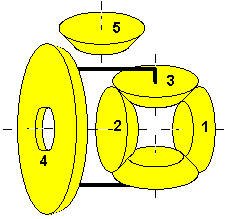If you look under the rear of a lorry you most probably will will see a thick "blob" in the middle of the rear axle, with three shafts entering to it: The axle to the left rear wheel, the axle to the right rear wheel and the drive shaft to somewhere in front of the axle. This "blob" contains nothing more than the differential described in the previous chapter plus one more bevel gear (5), which imparts the drive shaft's rotation to wheel 4, now also beveled. As we know by now, with a POV from right for wheel 4 and above for wheel 5 we can put down:
(h)    n4 * wrot4 - n5 * wrot5 = 0
Merging this with equation (D) from above, we get
(i)    rot1 + rot2 = 2 * (n5/n4) * rot5
For convenience the faktors preceding rot5 are combined to a parameter k, which is characteristic for each packaged differential.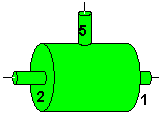(G)    rot1 + rot2 = k * rot5
In the plans on this site we will depict the packaged differential with the green symbol to the left.

## Mirrored Differential

Now one more mental exercise: If we flip wheel 4 to the other side of the construction, what does this mean to our mathematics? If we maintain our POV, only wheel 5 will change its sense of rotation. For differentials with this configuration we get the characteristic equation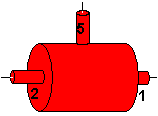(H)    rot1 + rot2 = - k * rot5
The red symbol to the left shall notify this mirrored or inverse differential.

## Some brain capacity left? Click here for the math of Planetary Gears!

To round off this introduction, some examples:

## Example 1: Idler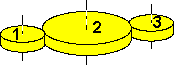Sometimes it is necessary to change the direction of a wheels rotation. This is easy to accomplish using an extra wheel (2) interposed between the wheel to change (3) and the driving wheel (1).
Using rule (1) from above - all shafts are fixed to the table -, we note:
n1 * wrot1 + n2 * wrot2 = 0
n2 * wrot2 + n3 * wrot3 = 0
Eliminating n2 (e.g. by subtracting the second line) results in
n1 * wrot1 - n3 * wrot3 = 0
or in other words, wheels 1 and 3 rotate in the same direction.
Another useful point to remember is that n2 (the size of wheel 2) is completely irrelevant. It can be chosen freely to best fit size and torque constraints.

## Example 2: Spur Wheel Differential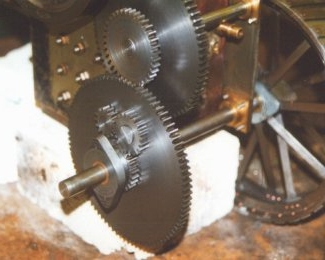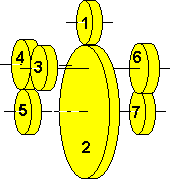The differential explained above uses bevel gears - which are expensive to make and even harder to get on short notice. Little wonder that the following design has some fans among steam tractor builders (See Ron Ginger's Minnie on the picture nearby)..

Wheels 3 and 6 are both fixed to a common shaft.

(a)    rot3 = rot6
Wheel 1 is the power input and meshes with transfer wheel 2, which runs freely on the axle.
(b)    n1 * wrot1 + n2 * wrot2 = 0
Both wheels' shafts are attached to the vehicle.
(c)    drot1 = drot2 = 0
Wheel 4 is an idler and the left road wheel is attached to wheel 5.
(d)    n5 * wrot5 - n3 * wrot3 = 0
The right road wheel is attached to wheel 7.
(e)    n7 * wrot7 + n6 * wrot6 = 0
And now the trick:  The shaft of wheels 3 and 6 is bushed in transfer wheel 2, so when wheel 2 turns with the shaft locked:
(f)    drot5 = drot7 = wrot2
The superposition rule (3) decrees:
(g)    rot5 = drot5 + wrot5
(h)    rot7 = drot7 + wrot7
Putting all this through the math mill gives:
(h:)    rot7 = drot7 + wrot7
(e:)    = drot7 - (n6/n7) * wrot6
(a:)    = drot7 - (n6/n7) * wrot3
(d:)    = drot7 - (n6/n7) * (n5/n3) * wrot5
(g:)    = drot7 - (n6/n7) * (n5/n3) * rot5 + (n6/n7) * (n5/n3) * drot5
(f:)    = wrot2 - (n6/n7) * (n5/n3) * rot5 + (n6/n7) * (n5/n3) * wrot2
= ( 1 + (n6/n7) * (n5/n3)) * wrot2 - (n6/n7) * (n5/n3) * rot5
(b:)    = (-1) * {( 1 + (n6/n7) * (n5/n3) *  (n1/n2) * wrot1 + (n6/n7) * (n5/n3) * rot5 }

(c:)    (n7/n6) * rot7 + (n5/n3) * rot5 + {(n7/n6) + (n5/n3)} * (n1/n2) * rot1 = 0

Setting all ratios to 1 results in
rot7 + rot5 + 2 * rot1 = 0
Sounds familiar? Don't worry about the sign mismatch to rule (4) - another spur wheel or starting at wheel 2 will fix this easily.
 Last not least it shall be mentioned that the spur wheel differential can be built "boxed" as well: The central drive wheel (2) is replaced by a drum enclosing the complete mechanism. (2) now being out of the way, wheels (3) and (6) can be merged. To cater for higher forces any number of pairs ((4),(3+6)) can be put into the box.